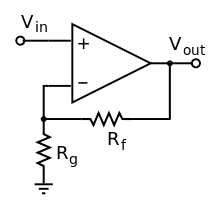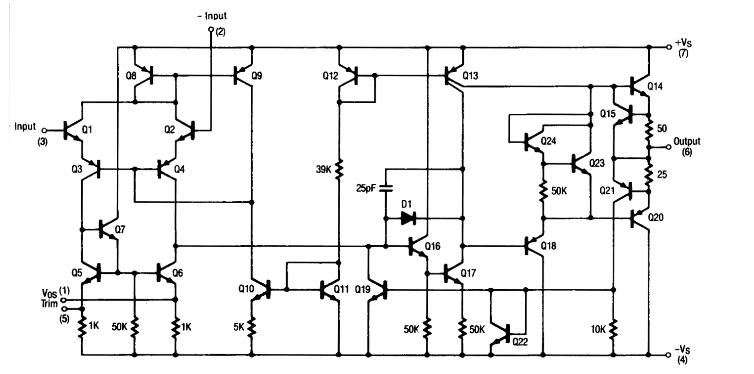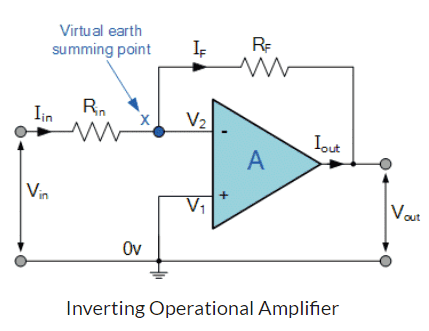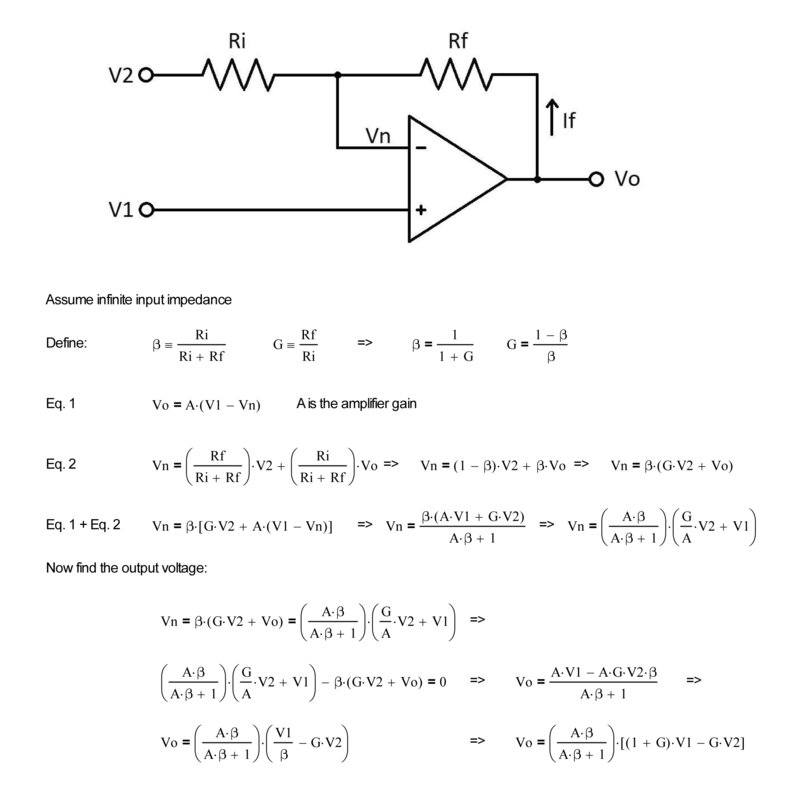# Understanding Op-Amp negative feedback

• I
Kaguro
TL;DR Summary
I think negative feedback should converge the inputs exactly same.
In an op-amp with negative feedback, say initially the + input has some small positive value and the - input is at 0. Then the op-amp amplifies this difference, and a part of it is fed to the - terminal through resistances. This will increase - and will lessen the difference.

My question is, why does this ever stop? I think this should go on until, the they are exactly 0, and then the output will become 0.

If this happens, and it happens quickly, how does an op-amp amplify any signal at all? It should always focus on keeping inputs same quickly leading to 0 output.

Homework Helper
Let's be specific. Say that "small positive value" is 1 millivolt supplied through a 1K resistor. And let's say that it is also tied to the Op Amp output through a 1K resistor.

So to balance it off, the output of the Op Amp would have to supply -1 millivolts.

But the open-loop differential gain for any is not infinite - and there could be practical problems if it were.
So, instead, the balance point is when something like 99.999% of that initial 1 millivolt has been eliminated.
At that point, the Op Amp is applying approximately -1 millivolts and the voltage difference between its inputs is -1 millivolts divided by the open-loop gain.

Just as an example, look at this data sheet:

It states the open look gain in terms of dB: "Open-loop differential voltage gain: 100 dB typical". That would represent a voltage increase of 100,000.

Kaguro
Let's be specific. Say that "small positive value" is 1 millivolt supplied through a 1K resistor. And let's say that it is also tied to the Op Amp output through a 1K resistor.

So to balance it off, the output of the Op Amp would have to supply -1 millivolts.

But the open-loop differential gain for any is not infinite - and there could be practical problems if it were.
So, instead, the balance point is when something like 99.999% of that initial 1 millivolt has been eliminated.
At that point, the Op Amp is applying approximately -1 millivolts and the voltage difference between its inputs is -1 millivolts divided by the open-loop gain.

Just as an example, look at this data sheet:

It states the open look gain in terms of dB: "Open-loop differential voltage gain: 100 dB typical". That would represent a voltage increase of 100,000.
You mean that there is a mechanism inside op amp which stops amplifying the difference in inputs when that difference is lower than a threshold value?

Gold Member
You mean that there is a mechanism inside op amp which stops amplifying the difference in inputs when that difference is lower than a threshold value?
No. Op-amps are assumed to be linear devices; their (idealized) behavior doesn't change.

Homework Helper
Gold Member
Summary:: I think negative feedback should converge the inputs exactly same. And you'd be right.

In an op-amp with negative feedback, say initially the + input has some small positive value and the - input is at 0. Then the op-amp amplifies this difference, and a part of it is fed to the - terminal through resistances. This will increase - and will lessen the difference.
It sounds to me as if you're confusing the virtual eath inverting amp with the non-inverting amp.

In the non-inverting amp, the inverting input is not tied directly to 0 V, it goes via a resistor which is part of the gain setting feedback.The - input is not at 0 V, but at a fraction of the output V determined by Rf and Rg. Vin is at roughly the same potential.
"say initially the + input has some small positive value" (which is higher than the - input), then the output will rise, increasing the voltage at -input until it again matches + input (Vin).
Then Vout = Vin x (Rg +Rf)/Rg (and V- = V+ = Vin)

My question is, why does this ever stop? I think this should go on until, the they are exactly 0, and then the output will become 0.
It goes on until the two inputs match, but they don't have to be at 0

Mentor
You mean that there is a mechanism inside op amp which stops amplifying the difference in inputs when that difference is lower than a threshold value?
No. Real world amplifiers have finite gain. That gain can be very high for optimized multi-stage amplifiers like you see in opamps, but it is still finite.

Here is an introduction to finite open loop gain: https://en.wikipedia.org/wiki/Open-loop_gain

And are you familiar with the transistor circuitry inside a typical opamp? That is the easiest way to see why the gain of real-world amplifier circuits is finite (and never infinite). The LM741, for example is one of the earliest opamps, and one of the easiest to understand, IMO. Here is a link to a good introduction, as well as the internal circuit of the LM741. Can you say a bit about what each stage of the opamp is doing, and what the gain of each stage is? And if you are familiar with the differences between Bipolar and CMOS Current Mirrors, can you say a bit about why higher-open-loop-gain opamps are often based on CMOS circuitry?http://www.righto.com/2015/10/inside-ubiquitous-741-op-amp-circuits.htmlMentor
BTW, here's a fun article about the birth of the Widlar-Fullagar LM741. Great stuff!https://spectrum.ieee.org/tech-hist...ll-of-fame-fairchild-semiconductor-a741-opamp

Fullagar took his design to the head of R&D at Fairchild, a guy named Gordon Moore, who sent it to the company’s commercial division. The new chip, the μA741, would become the standard for op-amps. The IC—and variants created by Fairchild’s competitors—have sold in the hundreds of millions. Now, for \$300—the price tag of that primordial 702 op-amp—you can get about 2,000 of today’s 741 chips.

•hutchphd, BvU and Merlin3189
Gold Member
You mean that there is a mechanism inside op amp which stops amplifying the difference in inputs when that difference is lower than a threshold value?
No. It's the negative sign of the feedback that achieves the (very nearly) zero difference between the two inputs. Without the feedback, an op amp will give an output voltage of nearly hard Max+ or Max-. What has been your source of information about this topic so far?

Homework Helper
Gold Member
...
My question is, why does this ever stop? I think this should go on until, they are exactly 0, and then the output will become 0.

If this happens, and it happens quickly, how does an op-amp amplify any signal at all? It should always focus on keeping inputs same quickly leading to 0 output.
YES It does try to keep both inputs the same, but NO that is not zero or ground.

Before anyone complains, not exactly the same because there may be an input offset voltage - in the 741 as much as 6 mV, though modern chips can be hundreds of times lower, MAX400 says 10 μV.

But your big problem is understanding the external circuit. An inverting amp can connect it's + input to ground/ Earth / zero and the - input will be maintained the same (or very close, as above) which is called a virtual earth. Then the input is applied to the - input via a resistor which is part of the gain-setting fb cct.EDIT: I missed one point. The output can be zero when both inputs are the same, even if that is both at 5V say. The output depends on the difference between the inputs. So though the effect of the external circuit is to minimise this difference, there must actually be a small difference for the output to be non-zero.

PS
Berkeman takes me back to 1973 in Dudley reference library poring over a 741 datasheet trying to figure out what on Earth an op-amp was and why, if it had a gain of 100,000, did we HAVE TO apply so much negative fb and end up with single figure gain.

Seeing your 741 post, I picked up my NS Linear Databook (1980) (still on my bookshelf! Why?) and it had two bookmarks (punch cards). The first was the 741, the second just over half an inch further on, was ?
555

•hutchphd and BvU
Gold Member
Summary:: I think negative feedback should converge the inputs exactly same.

If this happens, and it happens quickly, how does an op-amp amplify any signal at all?
It 'amplifies' the signal by chasing a potted down version of the output and applying it to the inverting input. The difference between the two op amp inputs will with be a tiny bit positive or a tiny bit negative (a sort of quasi-zero) which is just enough to raise or lower the output signal level to what it should be (= an amplified version of the input signal).

It's strange how everyone can appreciate how a thermostat works; there's something intuitive about the effect of a delay and a bit of hysteresis in that non linear (on/off) feedback system. If you just imagine a bimetal strip with a proportional output, you have the same situation as in an op amp.

Gold Member
While I'm a big fan of intuitive understanding, sometimes, for simple circuits, that is easiest to achieve after some analysis. This is a case where the answer is in the equations, and that analysis is pretty straight forward algebra. Although I will admit it's easier if you start out knowing what form the answer will be in.•sophiecentaur
Gold Member
The bottom line of the above bit of Maths is that when A approaches infinity (like the Op Amps you can buy today) and when the result ends up as
VO = V1(1-G) -V2G
and, when G is a lot bigger than1, you get
VO =(V2-V1)G
which is determined only by the values of the two resistors.

Gold Member
You mean VO = V1(1+G) -V2G and VO = (V1-V2)G
Anyway, this is an exact solution for this problem, you can use it as you wish. It is the basis for all Op-Amp negative feedback circuits.
Normally if you want a differential amp VO = (V1-V2)G, you would add a resistive divider in front of the + input to reduce that gain by G/(G+1). This turns out to be the same two resistor values used in the - side (actually, just the same ratio; just like a wheatstone bridge).
But there are other Diff Amp implementations too, typically with inverters and summers. For example, you may want the same input impedance in each input (an "Instrumentation Amp"). The simple circuit shown doesn't do that.

•sophiecentaur
Gold Member

Gold Member
Yes, the ideal Op-Amp solution would have A →∞

Homework Helper
Gold Member
From Dave's post,
##V_n = \left( \frac {A.\beta}{A.\beta +1}\right) \left( \frac G A . V_2 +V_1 \right) ##
which when A→∞ becomes ## V_n → 1 \left( 0 . V_2 +V_1 \right) \ \ ## or ##\ \ V_n → V_1 ##

Isn't that exactly what Kaguro said right at the beginning?
Summary:: I think negative feedback should converge the inputs exactly same.
Doesn't that suggest he knows this maths, but maybe is a bit short on the intuition about what it means?

Gold Member
Yes, I suppose so. But he also said "...and then the output will become 0" which is wrong, of course.

I guess it's about having the analysis background to describe how the feedback happens. If you mean he knows his maths in a computational sense, OK. I think constructing two equations based on a simple circuit is more rote application of basic electronics than intuition.

But I must confess, I learned about feedback and circuit analysis in a more rigorous, theoretical way. So I know I am not a great "touchy-feely" instructor for electronics, that sort of approach stops working pretty quickly when things get harder. In my world you should learn rigorous solutions to the simplest problems and then develop the intuition to apply those to simplify the more complex scenarios.

I suppose for something abstract, like circuits, it's a chicken and egg problem: analysis leads to insight, insight often makes analysis easier.

•sophiecentaur
Gold Member
Another thing to consider is the input impedance of real amplifiers is not infinite. Some small amount of current can bleed out the input leading to some additional (and unsuspected) voltage drops. The size of this current depends quite strongly on the design of the input stage of the chip. Many opamps need to be and have offset balance pins for this purpose.

•